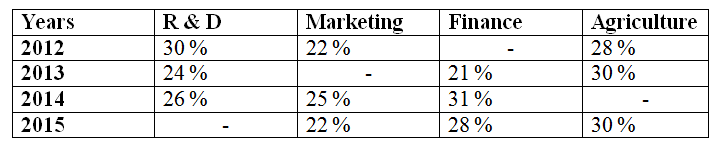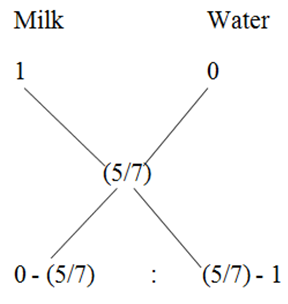# IBPS PO Mains 2018 – Quantitative Aptitude Questions Day-23

Dear Readers, Bank Exam Race for the Year 2018 is already started, To enrich your preparation here we have providing new series of Practice Questions on Quantitative Aptitude – Section. Candidates those who are preparing for IBPS PO Mains 2018 Exams can practice these questions daily and make your preparation effective.

[WpProQuiz 4238]

1) Mano sold a book at a loss of 13 %. If he had sold that book for Rs. 84 more, he would have gained 15 %. Find the selling price of the book to get a profit of 20 %?

a) Rs. 360

b) Rs. 420

c) Rs. 480

d) Rs. 500

e) None of these

2) A certain sum is divided among A, B and C in such a way that, A gets 120 more than two-fifth of the sum. B gets 190 less than one-fourth of the sum and C gets Rs. 420. What is the 65 % of the total sum?

a) Rs. 650

b) Rs. 500

c) Rs. 400

d) Rs. 525

e) None of these

3) A can do 1/3 of the work in 5 days, B can do 2/5 of the work in 10 days. Then in how many days both of them together can do the work?

a) 7 ¾ days

b) 8 5/6 days

c) 10 7/9 days

d) 9 3/8 days

e) None of these

4) The simple interest accrued on an amount of Rs. 15,000 at the end of two years is Rs. 2400. What would be corresponding Compound Interest?

a) Rs. 2496

b) Rs. 2512

c) Rs. 2538

d) Rs. 2474

e) None of these

5) In what ratio water be mixed with milk to earn a profit of 40 % by selling the mixture at cost price?

a) 7 : 4

b) 5 : 2

c) 6 : 5

d) 2 : 7

e) None of these

Directions (Q. 6 – 10) Study the following information carefully and answer the given questions:

The following table shows the percentage distribution of expenses of four departments in 4 different years.Total expenses of these 4 departments

In the year 2012 = 60 lakhs

In the year 2013 = 68 lakhs

In the year 2014 = 72 lakhs

In the year 2015 = 75 lakhs

Note:

Profit = Income – Expense

Profit % = (Profit/Expense)*100

6) If the profit % of Finance department in the year 2012 is 20 %, then find the total income of finance department in the year 2012?

a) 15.2 lakhs

b) 14.4 lakhs

c) 13.8 lakhs

d) 16.4 lakhs

e) None of these

7) Find the difference between the total profit earned by marketing department in the year 2013 to that of total expenses of R & D department in the year 2014 and 2015 together, if the profit % of marketing department in the year 2013 is 15 %?

a) 2895000

b) 2913000

c) 3056000

d) 3117000

e) None of these

8) Find the average expenses of agriculture in all the given years together?

a) 1816500

b) 1578200

c) 1649400

d) 1752600

e) None of these

9) If the profit earned by marketing department and R & D department in the year 2014 and 2015 respectively is 4.5 lakhs and 3 lakhs, then find the profit % of marketing department is what percentage of profit % of R & D department?

a) 110 %

b) 135 %

c) 125 %

d) 100 %

e) 90 %

10) Find the ratio between the total expenses of marketing and agriculture department in the year 2012 to that of total expenses of agriculture department in the year 2015?

a) 4 : 3

b) 5 : 2

c) 11 : 9

d) 23 : 17

e) None of these

Let the cost price be Rs. X,

X*(87/100) + 84 = x*(115/100)

(115x/100) – (87x/100) = 84

28x/100 = 84

X = 84*(100/28)

X = 300

Cost price = Rs. 300

Selling price = 300*(120/100) = Rs. 360

Total sum = X = A + B + C

A= (2/5)*X + 120

B = X/4 – 190

C = 420

Total sum(X) = (2X/5) + 120 + (X/4) – 190 + 420

X= (8X + 5X)/20 + 350

X – (13x/20) = 350

7x/20 = 350

X = 1000

65 % of the total sum

= > 1000*(65/100) = Rs. 650

A can do the work in,

= > (1/3)*work = 5

= > Whole work = 15 days

B can do the work in,

= > (2/5)*work = 10

= > Whole work = 25 days

(A + B)’s one day work = (1/15) + (1/25) = 40/(15*25) = 8/75

(A + B) can do the work in, 75/8 = 9 3/8 days

S.I = PNR/100

2400 = (15000*2*R)/100

R = (2400*100)/30000

R = 8 %

Corresponding CI:

15000*(8/100) = 1200

16200*(8/100) = 1296

C.I = 1200 + 1296 = Rs. 2496

Let the cost price be Rs. 1,

Selling price of mixture = Rs. 1

Cost price of mixture = 1*100/140 = 5/7= > – (5/7) : – (2/7)

= > 5 : 2

Direction (6-10) :

Total expenses of 4 departments in the year 2012 = 60 lakhs

Total expenses of finance department in the year 2012

= > 60*(20/100) = 12 lakhs

Profit % = 20 %

Total income of finance department in the year 2012

= > Income = 12*(120/100)

= > Income = 14.4 lakhs

The total profit earned by marketing department in the year 2013

Expense of marketing in the year 2013 = 68*(25/100) = 17 lakhs

Income of marketing in the year 2013 = 17*(115/100) = 19.55 lakhs

Profit = 2.55 lakhs

Total expenses of R & D department in the year 2014 and 2015 together

= > 72*(26/100) + 75*(20/100)

= > 18.72 + 15 = 33.72 lakhs

Required difference = 33.72 – 2.55 = 31.17 lakhs = 3117000

The total expenses of agriculture in all the given years together

= > 60*(28/100) + 68*(30/100) + 72*(18/100) + 75*(30/100)

= > 16.8 + 20.4 + 12.96 + 22.5

= > 72.66 lakhs

Required average = 72.66/4 = 18.165 lakhs = 1816500

The profit earned by marketing department the year 2014 = 4.5 lakhs

Expense of marketing department the year 2014 = 72*(25/100) = 18 lakhs

Profit % of marketing department the year 2014 = (4.5/18)*100 = 25 %

The profit earned by R & D department the year 2015 = 3 lakhs

Expense of R & D department the year 2015 = 75*(20/100) = 15 lakhs

Profit % of R & D department the year 2015 = (3/15)*100 = 20 %

Required % = (25/20)*100 = 125 %

The total expenses of marketing and agriculture department in the year 2012

= > 60*(50/100) = 30 lakhs

The total expenses of agriculture department in the year 2015

= > 75*(30/100) = 22.5 lakhs

Required ratio = 30 : 22.5 = 300 : 225 = 4 : 3

Daily Practice Test Schedule | Good Luck

 Topic Daily Publishing Time Daily News Papers & Editorials 8.00 AM Current Affairs Quiz 9.00 AM Current Affairs Quiz (Hindi) 9.30 AM IBPS Clerk Prelims – Reasoning 10.00 AM IBPS Clerk Prelims – Reasoning (Hindi) 10.30 AM IBPS Clerk Prelims – Quantitative Aptitude 11.00 AM IBPS Clerk Prelims – Quantitative Aptitude (Hindi) 11.30 AM Vocabulary (Based on The Hindu) 12.00 PM IBPS PO Prelims – English Language 1.00 PM SSC Practice Questions (Reasoning/Quantitative aptitude) 2.00 PM IBPS PO/Clerk – GK Questions 3.00 PM SSC Practice Questions (English/General Knowledge) 4.00 PM Daily Current Affairs Updates 5.00 PM IBPS PO Mains – Reasoning 6.00 PM IBPS PO Mains – Quantitative Aptitude 7.00 PM IBPS PO Mains – English Language 8.00 PM